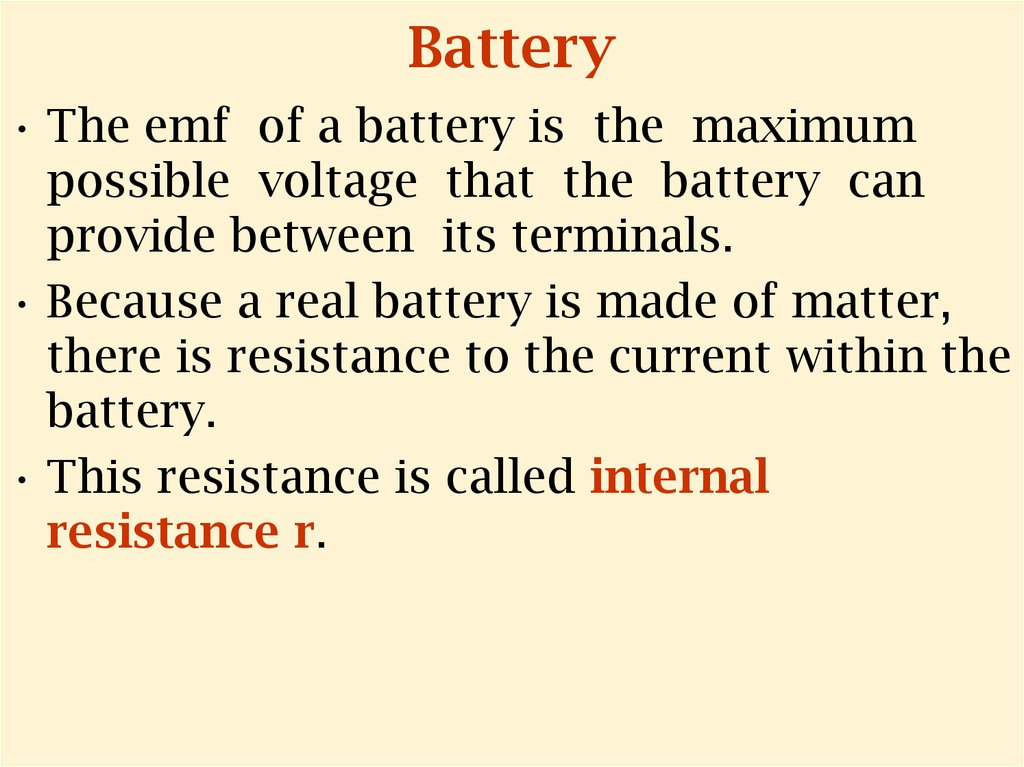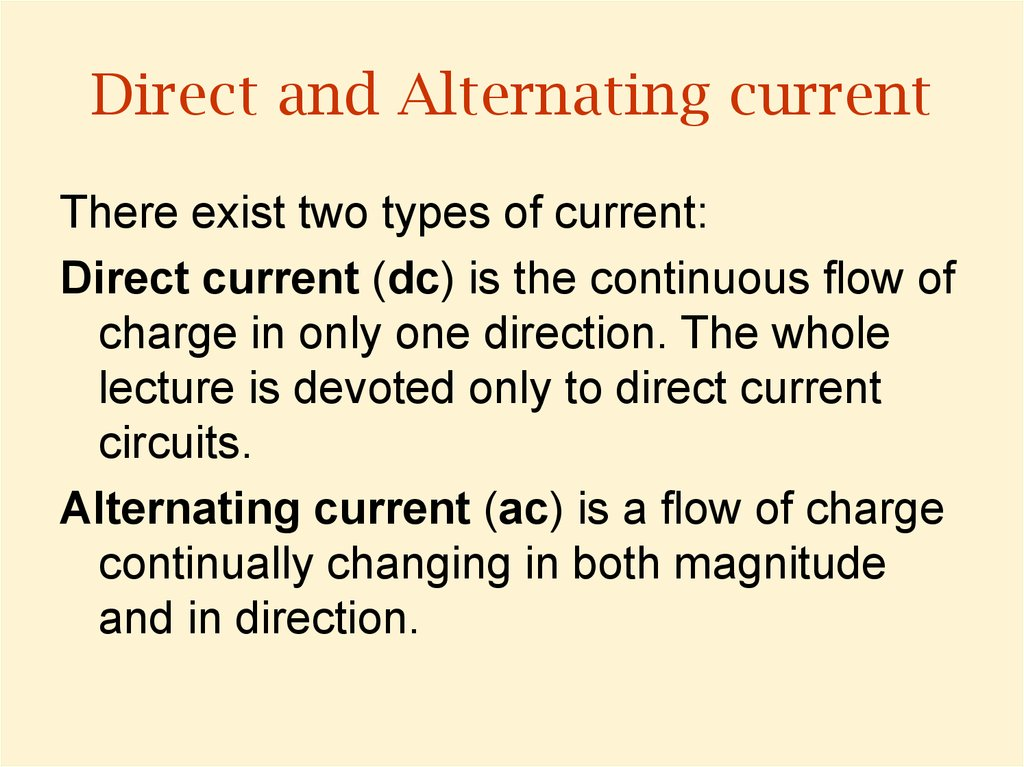# Battery. Direct and Alternating current

## 1.

Republic of Kazakhstan
Ministry of Education and Science
Kazakh-British Technical University
Faculty of Power and Oil and Gas Industry
Physical Engineering Department
Physics 1

## 2. Battery

• The emf of a battery is the maximum
possible voltage that the battery can
provide between its terminals.
• Because a real battery is made of matter,
there is resistance to the current within the
battery.
• This resistance is called internal
resistance r.

## 3. Direct and Alternating current

There exist two types of current:
Direct current (dc) is the continuous flow of
charge in only one direction. The whole
lecture is devoted only to direct current
circuits.
Alternating current (ac) is a flow of charge
continually changing in both magnitude
and in direction.

## 4.

- emf
• V – potential
difference on the
battery ( V= Vb-Va)
• r – internal
resistance of emf
Vb-Va:
Circuit current:
Power output of the
battery is *I:
V= - IR
I= /(R+r)
*I = I2R + I2r

## 5. Energy output of a Battery

*I = I2R + I2r
• *I - Power output of the battery.
• I2R – energy transferred to the external load
• I2r – energy loss by the internal resistance
• So the power output of the battery to
external resistance is accompanied by the
power loss due to internal resistance.

## 6. Resistor

• Resistor is a circuit element which is
used to control the current level in the
various parts of the circuit. It’s main
property – it has constant resistivity
for a wide range of potential
differences.

## 7. Resistors in Series

• Iac=I1=I2
• Vac=V1 + V2
• Rac=R1 + R2
• Currents I1 and I2 are the same in both
resistors because the amount of charge
that passes through must also pass
through in the same time inter interval.

## 8. Resistors in Parallel

• I=I1+I2
• Vac=V1=V2
• When resistors are connected in parallel, the
potential differences across the resistors are
the same.

## 9. Any number of resistors

• In series:
I=I1=I2=I3=…
V=V1 + V2 + V3 + …
Rac=R1 + R2 + R3 + …
• In parallel:
I=I1 + I2 + I3+ …
V=V1 = V2 = V3 = …

## 10. Kirchhoff’s Rules for Direct Current Circuits

1. Junction rule. The sum of the currents
entering any junction in a circuit must
equal the sum of the currents leaving
that junction.
2. Loop rule. The sum of the potential
differences across all elements around
any closed circuit loop must be zero.

## 11. Junction Rule

• I1= I2 + I3
• The Kirchhoff’s
junction rule is an
analogue for fluid
current.
• The junction rule is a
consequence of the
Charge conservation
law.

## 12. Loop Rule Basis

• Kirchhoff’s second rule follows from the law of
conservation of energy. Let us imagine moving a charge
around a closed loop of a circuit. When the charge returns
to the starting point, the charge –circuit system must have
the same total energy as it had before the charge was
moved. The sum of the increases in energy as the charge
passes through some circuit elements must equal the sum
of the decreases in energy as it passes through other
elements.
• The potential energy decreases whenever the charge
moves through a potential drop -IR across a resistor or
whenever it moves in the reverse direction through a
source of emf. The potential energy increases whenever
the charge passes through a battery from the negative
terminal to the positive terminal.

## 13. Loop rule

In Figures a-d each
element is traversed
from left to right.
• If a resistor is traversed in the direction of
the current, the potential difference across
the resistor –IR. (Fig. a)
• If a resistor is traversed in the direction
opposite the current, the potential differdifference the resistor is +IR. (Fig. b)
• If a source of emf (assumed to have zero
internal resistance) is traversed in the
direction of the emf (from - to +), the
potential difference is + . The emf of the
battery increases the electric potential as
we move through it in this direction. (Fig. c)
• If a source of emf (assumed to have zero
internal resistance) is traversed in the
direction opposite the emf (from + to - ), the
potential difference - . In this case the emf
of the batter battery reduces the electric
potential as we move through it. (Fig. c)

## 14. Kirchhoff’s rules validity

• Kirchhoff’s rules are valid only for steadystate conditions - that is, the currents in
various branches are constant.
• Any capacitor acts as an open branch in a
circuit; that is, the current in the branch
containing the capacitor is zero under

## 16. Example: a multiloop circuit

Given:
state, I3=50mA,
=6V,
R1=100 W,
R2= 80 W,
C=2mF.
Find:
I1, I2, R3, VC.
VC is the capacitor’s voltage
All currents are steady state means that there is no changes
in currents. In steady-state condition the capacitor acts as
an open switch despite the fact that it has voltage.

## 17.

I3=50mA,
=6V,
R1=100 W,
R2= 80 W,
C=2mF.
I1, I2, R3, VC= ?
So first we choose directions in the two circuits as it shown
in the picture.
I2=0, as the capacitor is not charging. =>
=> For junction b:
I3=I1.
For loop 1:
- I3R3 - I1R1 =>
R3= /I1 - R1
For loop 2:
- I3R3 -VC = 0 =>
VC= - I3R3 = - I3R3= I1R1

## 18. Units in Si

• Capacitance
• Current
• Resistance
• Electro motive force (emf)
C
I
R
F=C/V
A=C/s
Ohm=V/A
V